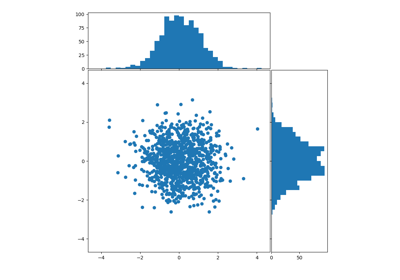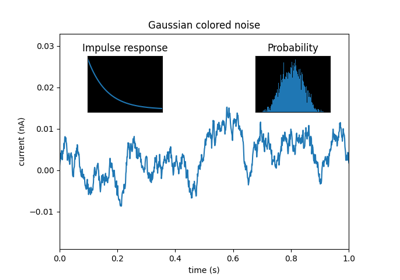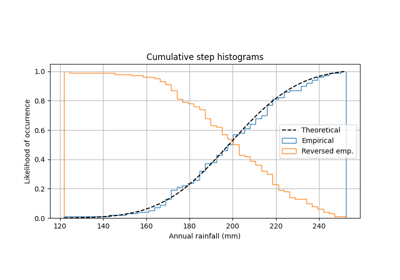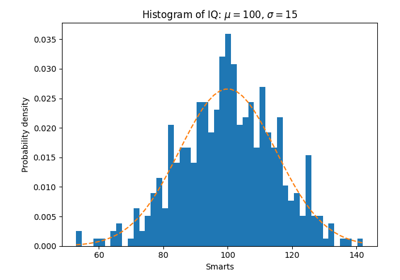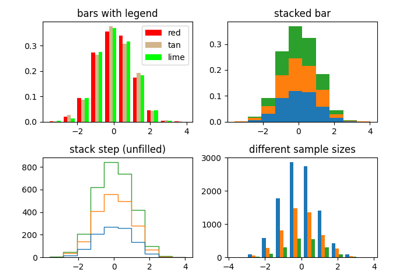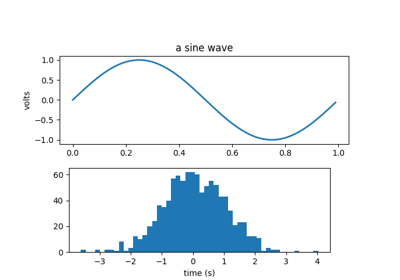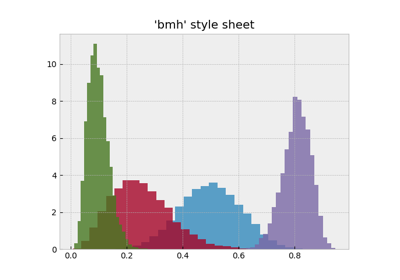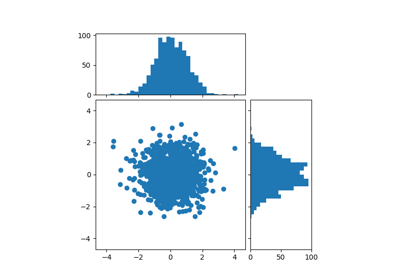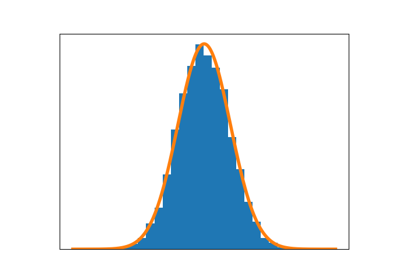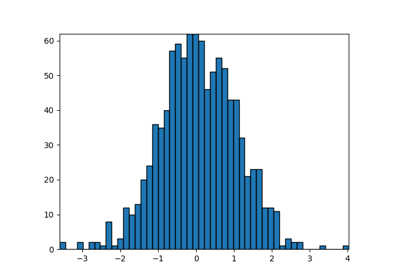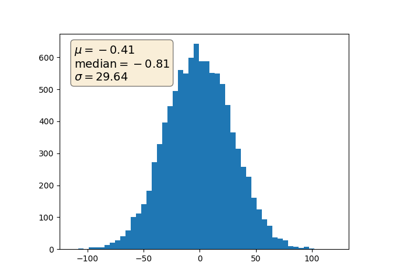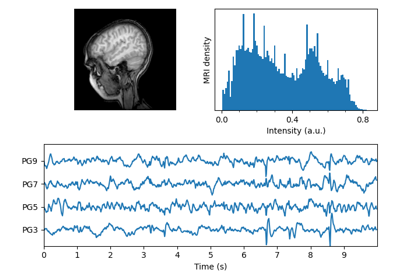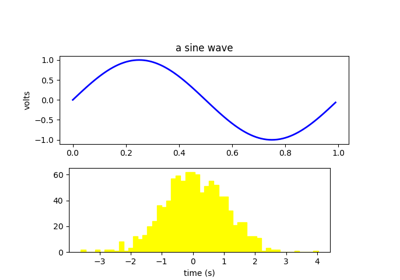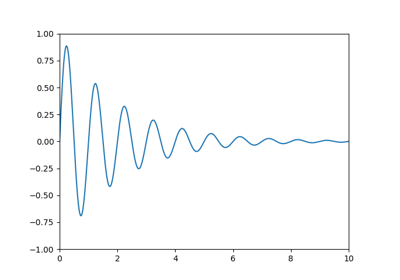# matplotlib.axes.Axes.hist¶

Axes.hist(self, x, bins=None, range=None, density=False, weights=None, cumulative=False, bottom=None, histtype='bar', align='mid', orientation='vertical', rwidth=None, log=False, color=None, label=None, stacked=False, \*, data=None, \*\*kwargs)[source]

Plot a histogram.

Compute and draw the histogram of x. The return value is a tuple (n, bins, patches) or ([n0, n1, ...], bins, [patches0, patches1,...]) if the input contains multiple data. See the documentation of the weights parameter to draw a histogram of already-binned data.

Multiple data can be provided via x as a list of datasets of potentially different length ([x0, x1, ...]), or as a 2-D ndarray in which each column is a dataset. Note that the ndarray form is transposed relative to the list form.

The bins, range, weights, and density parameters behave as in numpy.histogram.

Parameters: x(n,) array or sequence of (n,) arraysInput values, this takes either a single array or a sequence of arrays which are not required to be of the same length. binsint or sequence or str, optionalIf bins is an integer, it defines the number of equal-width bins in the range. If bins is a sequence, it defines the bin edges, including the left edge of the first bin and the right edge of the last bin; in this case, bins may be unequally spaced. All but the last (righthand-most) bin is half-open. In other words, if bins is: [1, 2, 3, 4]  then the first bin is [1, 2) (including 1, but excluding 2) and the second [2, 3). The last bin, however, is [3, 4], which includes 4. If bins is a string, it is one of the binning strategies supported by numpy.histogram_bin_edges: 'auto', 'fd', 'doane', 'scott', 'stone', 'rice', 'sturges', or 'sqrt'. The default is rcParams["hist.bins"] (default: 10). rangetuple or None, optionalThe lower and upper range of the bins. Lower and upper outliers are ignored. If not provided, range is (x.min(), x.max()). Range has no effect if bins is a sequence. If bins is a sequence or range is specified, autoscaling is based on the specified bin range instead of the range of x. Default is None densitybool, optionalIf True, the first element of the return tuple will be the counts normalized to form a probability density, i.e., the area (or integral) under the histogram will sum to 1. This is achieved by dividing the count by the number of observations times the bin width and not dividing by the total number of observations. If stacked is also True, the sum of the histograms is normalized to 1. Default is False. weights(n, ) array-like or None, optionalAn array of weights, of the same shape as x. Each value in x only contributes its associated weight towards the bin count (instead of 1). If normed or density is True, the weights are normalized, so that the integral of the density over the range remains 1. Default is None. This parameter can be used to draw a histogram of data that has already been binned, e.g. using np.histogram (by treating each bin as a single point with a weight equal to its count) counts, bins = np.histogram(data) plt.hist(bins[:-1], bins, weights=counts)  (or you may alternatively use bar()). cumulativebool or -1, optionalIf True, then a histogram is computed where each bin gives the counts in that bin plus all bins for smaller values. The last bin gives the total number of datapoints. If density is also True then the histogram is normalized such that the last bin equals 1. If cumulative is a number less than 0 (e.g., -1), the direction of accumulation is reversed. In this case, if density is also True, then the histogram is normalized such that the first bin equals 1. bottomarray-like, scalar, or None, default: NoneLocation of the bottom of each bin, ie. bins are drawn from bottom to bottom + hist(x, bins) If a scalar, the bottom of each bin is shifted by the same amount. If an array, each bin is shifted independently and the length of bottom must match the number of bins. If None, defaults to 0. histtype{'bar', 'barstacked', 'step', 'stepfilled'}, optionalThe type of histogram to draw. 'bar' is a traditional bar-type histogram. If multiple data are given the bars are arranged side by side. 'barstacked' is a bar-type histogram where multiple data are stacked on top of each other. 'step' generates a lineplot that is by default unfilled. 'stepfilled' generates a lineplot that is by default filled. Default is 'bar' align{'left', 'mid', 'right'}, optionalControls how the histogram is plotted. 'left': bars are centered on the left bin edges. 'mid': bars are centered between the bin edges. 'right': bars are centered on the right bin edges. Default is 'mid' orientation{'horizontal', 'vertical'}, optionalIf 'horizontal', barh will be used for bar-type histograms and the bottom kwarg will be the left edges. rwidthscalar or None, optionalThe relative width of the bars as a fraction of the bin width. If None, automatically compute the width. Ignored if histtype is 'step' or 'stepfilled'. Default is None logbool, optionalIf True, the histogram axis will be set to a log scale. If log is True and x is a 1D array, empty bins will be filtered out and only the non-empty (n, bins, patches) will be returned. Default is False colorcolor or array-like of colors or None, optionalColor or sequence of colors, one per dataset. Default (None) uses the standard line color sequence. Default is None labelstr or None, optionalString, or sequence of strings to match multiple datasets. Bar charts yield multiple patches per dataset, but only the first gets the label, so that the legend command will work as expected. default is None stackedbool, optionalIf True, multiple data are stacked on top of each other If False multiple data are arranged side by side if histtype is 'bar' or on top of each other if histtype is 'step' Default is False narray or list of arraysThe values of the histogram bins. See density and weights for a description of the possible semantics. If input x is an array, then this is an array of length nbins. If input is a sequence of arrays [data1, data2, ...], then this is a list of arrays with the values of the histograms for each of the arrays in the same order. The dtype of the array n (or of its element arrays) will always be float even if no weighting or normalization is used. binsarrayThe edges of the bins. Length nbins + 1 (nbins left edges and right edge of last bin). Always a single array even when multiple data sets are passed in. patcheslist or list of listsSilent list of individual patches used to create the histogram or list of such list if multiple input datasets. **kwargsPatch properties

hist2d
2D histograms

Notes

Note

In addition to the above described arguments, this function can take a data keyword argument. If such a data argument is given, the following arguments are replaced by data[<arg>]:

• All arguments with the following names: 'weights', 'x'.

Objects passed as data must support item access (data[<arg>]) and membership test (<arg> in data).

## Examples using matplotlib.axes.Axes.hist¶